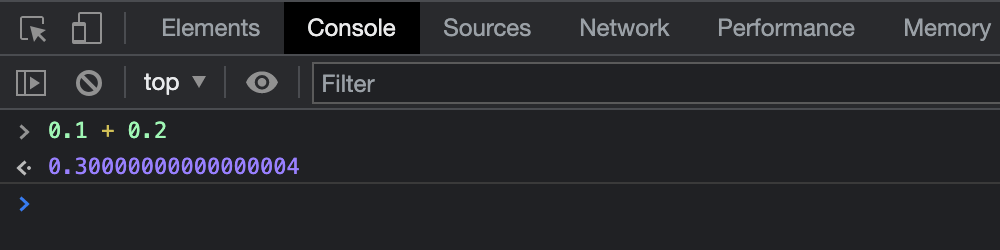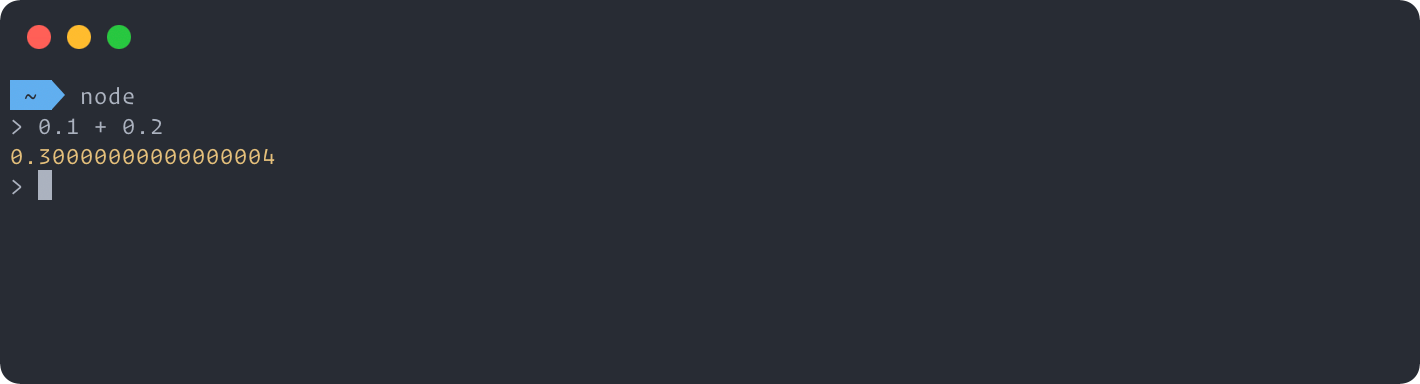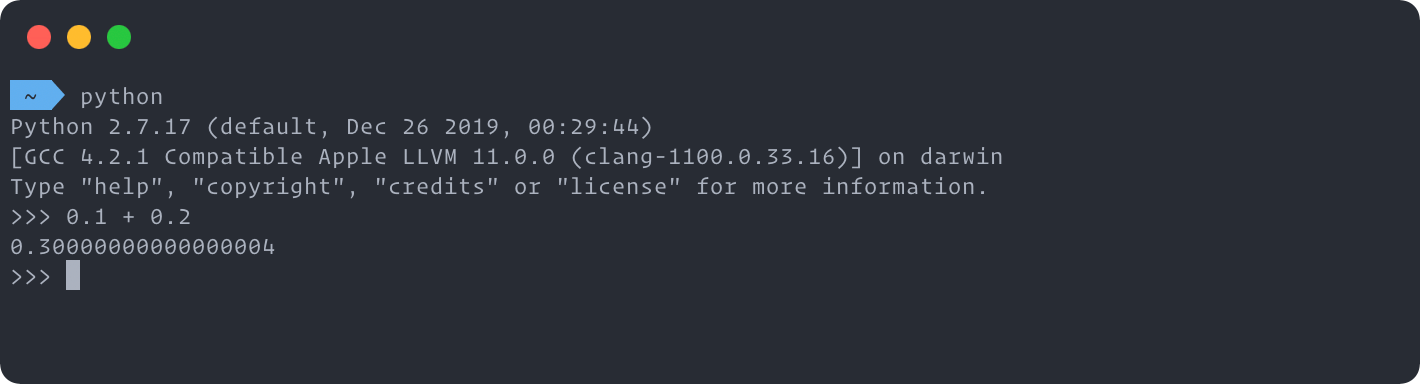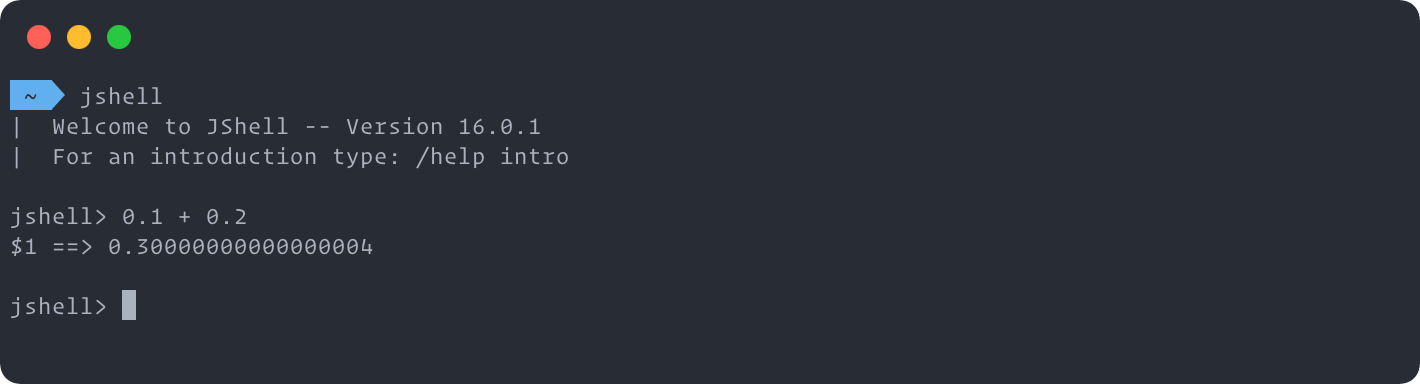# Why »0.1 + 0.2« results in »0.30000000000000004« in JavaScript

Anyone who wants to perform arithmetic operations with JavaScript will sooner or later encounter inaccurate results. To understand why, you need to know how numbers work in JavaScript, what floating-point math is, and how to circumvent this.

JavaScript has two number types: `Number` and `BigInt`, while `Number` is the most frequently-used one. (Alright, for the sake of completeness, there’s a proposal for `BigDecimal` as a pendant to `BigInt` in the making.)

That `Number` type is a 64-bit floating-point data type. Those 64 bits are divided according to the IEEE 754 standard (or »double-precision floating-point format«) so that bits `0` to `51` are used for the mantissa (i.e. the actual number value), bits `52` to `62` for the exponent, and the last bit (`63`) for the sign (i.e. whether the number is positive or negative).

• Mantissa = 52 bits (`0` to `51`) → digits of a number
• Exponent = 11 bits (`52` to `62`) → indicates where the point is
• Sign = 1 bit (`63`) → if the sign bit is `0`, the number is positive, otherwise it’s negative

This takes 8 bytes – or 8 bytes × 8 bits per byte = 64 bits – in a computer’s memory. Programming languages simply have a limited amount of memory and need to make a trade-off between range of numbers that can be represented and the precision of these numbers.

Each number is mapped in a binary exponential representation. For integer values, this means that a safe representation is possible up to 15 digits before rounding errors occur due to the exponential mapping.

``````const a = 999999999999999; // = 999999999999999
const b = 9999999999999999; // = 10000000000000000
``````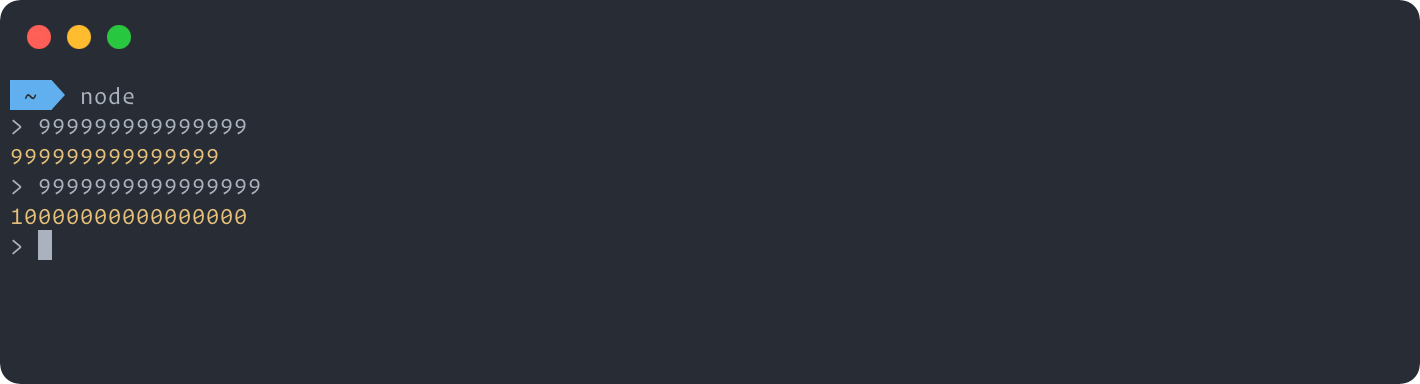Knowing that `Number.MAX_SAFE_INTEGER` (which is `9007199254740991`, the largest number that JavaScript can represent with `Number`) can be calculated via 253 - 1, you may recognize the 53 in that formula and ask yourself whether/how it’s related to the 52 bits of aforementioned mantissa.

Integers can be represented with the exponent being set in a way that the most significant bit (sometimes called »hidden bit«, »leading bit«, or »assumed bit«) is always `1` and therefore not stored, providing that 53rd bit of precision by shifting the mantissa, allowing holding up to 253 - 1 integers while there’s a special value to represent zero.

For floating-point numbers, this has a corresponding effect on the precision of the decimal places. Numbers can have a maximum of 16 numbers after the decimal point. Actually, it’s log10(2n) where n is 53, resulting in ~15.95 (which subsequently is rounded to 16 here).

It’s called »floating point« in general because there is no fixed number of digits before or after the decimal point, allowing it to represent a wide range of numbers both big and small.

## But where is the »0.30000000000000004« coming from?

It’s simply how floating-point numbers and the IEEE 754 standard work: you can’t represent an infinite number of real numbers in a finite number of bits. IEEE 754 is actually some kind of compression algorithm that allows to compress an infinite set of numbers from `0` to `Infinity` and from `0` to `-Infinity` and all the numbers that come in-between every one of those single integers.

And while the standard tries to capture/cover as many numbers as possible while keeping things sensible, no matter how many bits there are, there are always going to be gaps and some truncation because we simply don’t have an infinite amount of space available.

When the code is compiled or interpreted, `0.1` is already rounded to the nearest number in that format, resulting in a small rounding error even before the calculation happens. `0.1 + 2 - 2` visualizes this. So the computer never »sees« `0.1` but the best approximation it can get.

What is happening are the following steps.

• Converting `0.1` to the nearest value representable in the `Number` type (i.e. `0.1000000000000000055511151231257827021181583404541016`)
• Converting `0.2` to the nearest value representable in the `Number` type (i.e. `0.2000000000000000111022302462515654042363166809082031`)
• Adding the above two values and rounding the result to the nearest value representable in the `Number` type

Thus, `0.30000000000000004` is actually `0.3000000000000000444089209850062616169452667236328125` but has just enough significant digits to uniquely distinguish the value from its neighboring values.

``````0.299999999999999988897769753748434595763683319091796875
0.3000000000000000444089209850062616169452667236328125
0.300000000000000099920072216264088638126850128173828125
``````

You can verify this by running `(0.1 + 0.2).toPrecision(52)` as the `.toPrecision()` method allows to request more (or fewer) digits.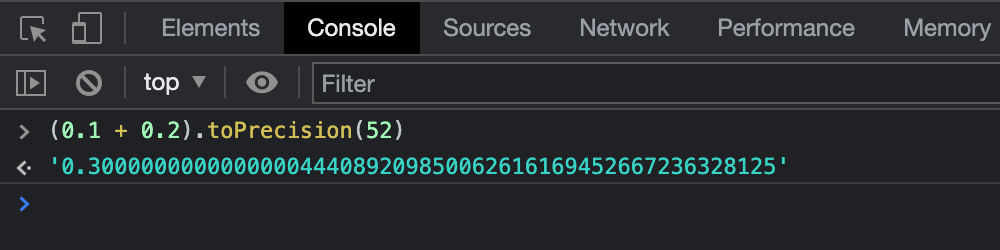## Well, why does »0.6 / 2« and »(1 + 2) / 10« work then?

Most decimal fractions can’t be represented exactly in binary floating-point: neither `0.1` nor `0.2` are. But for some values, the deviation from the actual value is usually too small to be displayed. For cases such as `0.1 + 0.3`, the result isn’t really `0.4` but close enough that `0.4` is the shortest number that is closer to the result than to any other floating-point number. JavaScript then displays that number `0.4` instead of converting the actual result back to the closest decimal fraction.

Remember: even though a printed value/result may look like an exact value, the actual stored value still is the nearest representable binary fraction.

## Are there other cases?

Yes, there are several other »storybook examples« where this can be observed.

• `0.1 + 0.7` = `0.7999999999999999`
• `0.4 + 0.2` = `0.6000000000000001`

## How to avoid those rounding errors?

For common use, it’s usually sufficient to limit yourself to 3-4 digits after the decimal point and use the native `.toPrecision()` or `.toFixed()` methods in JavaScript. The difference between these two is that `.toPrecision()` formats to a number of given significant digits while `.toFixed()` allows to specify the number of post-decimal digits to be represented.

``````(0.1 + 0.2).toPrecision(2) // = '0.30'
(0.1 + 0.2).toFixed(2) // = '0.30'
``````
``````(0.3).toPrecision(2) // = '0.30'
(0.3).toFixed(2) // = '0.30'
``````

Remember: As `.toPrecision()` formats to a number of given significant digits, this means it will not round to a total amount of post-decimal digits but to the significant (i.e. distinguishing) post-decimal digits as the following example showcases.

``````(0.000003).toPrecision(2) // = '0.0000030'
(0.000003).toFixed(2) // = '0.00'
``````

So both methods are named quite descriptive, actually. And both `.toFixed()` and `.toPrecision()` don’t return a number object but as `String` data type. Otherwise, again, it would not be possible to represent the number as `Number` data type.

If you’re forced to calculate (big) numbers within JavaScript, you might want to have a look at the built-in `BigInt` and the proposal for `BigDecimal` or use libraries such as bignumber.js or big.js.

## Why is it relevant?

Don’t use plain `Number` for situations where you need accuracy such as displaying prices/currencies, transactions, or quantities. If you really need/want to do this, make use of aforementioned ways how to properly round/represent decimals in JavaScript.

Otherwise, you may have a bad time with people having a balance of `0.30000000000000004` or `0.7999999999999999`.

## JavaScript is not alone

If you now feel confirmed that JavaScript is screwed-up and a toy language and can’t even do math properly, you’ll be surprised to learn that this exact problem is not limited to JavaScript but instead quite common across programming languages. Basically, all languages that implement floating-point numbers according to IEEE 754 have corresponding rounding errors.